Matlab之山东大学数字图像处理实验一（图像加载，显示与合成）

• 利用图像库的功能，实现从文件加载图像，并在窗口中进行显示的功能；利用常见的图像文件格式（.jpg; .png; .bmp; .gif）进行测试；

• 现有一张4通道透明图像a.png:
• 从其中提取出alpha通道并显示;
• 用alpha混合，为a.png替换一张新的背景（背景图自选）;

img = imread('a.bmp');
imshow(img)


%gif图像的特殊处理
for i=1:size(I,4)
imshow(I(:,:,:,i),map);
pause(0.001);
end


a.gif是以下这张图像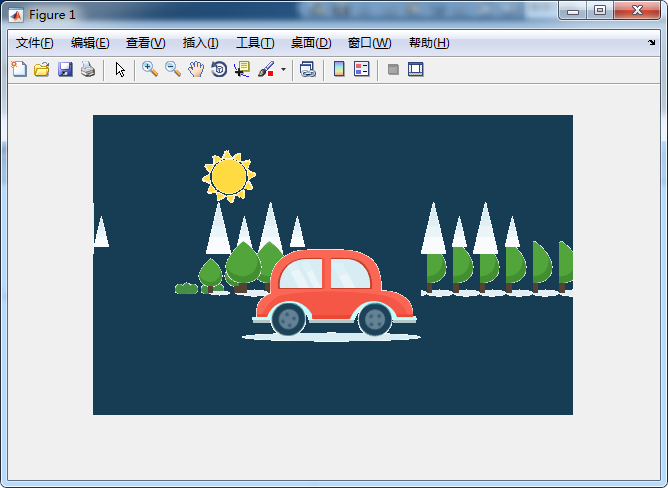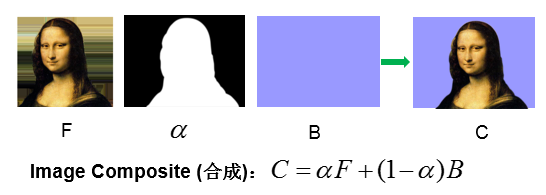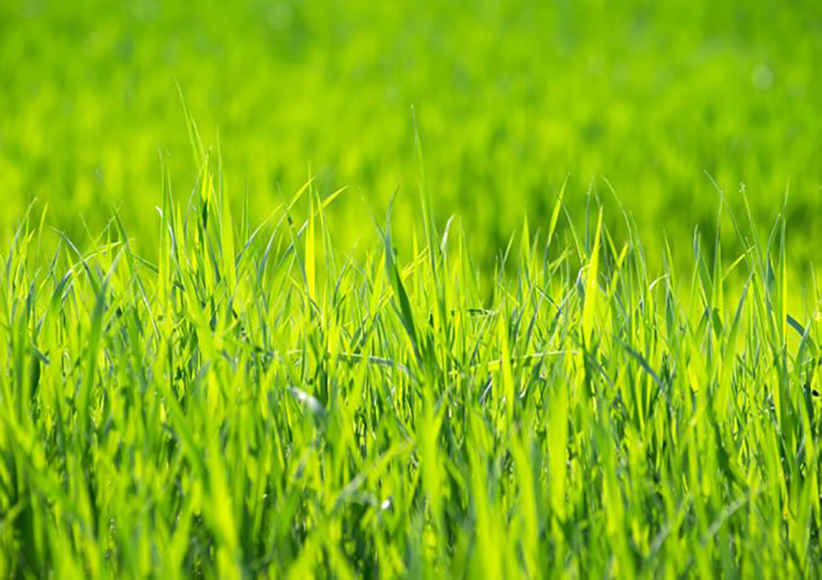img = imread('b.png');
%R通道
R = img(:,:,1);
%G通道
G = img(:,:,2);
%B通道
B = img(:,:,3);
%Alpha通道
%计算参数
a = Alpha/255;
%三通道合成
img2(:,:,1) = img(:,:,1) .* a + (1-a) .* background(:,:,1);
img2(:,:,2) = img(:,:,2) .* a + (1-a) .* background(:,:,2);
img2(:,:,3) = img(:,:,3) .* a + (1-a) .* background(:,:,3);
imshow(img2);
imwrite(img2,'combineB.png')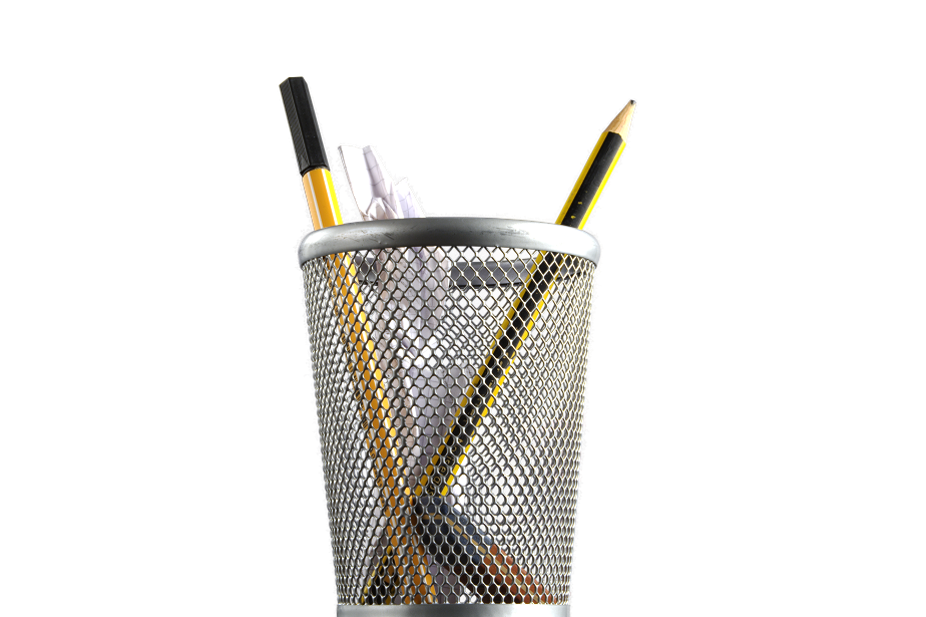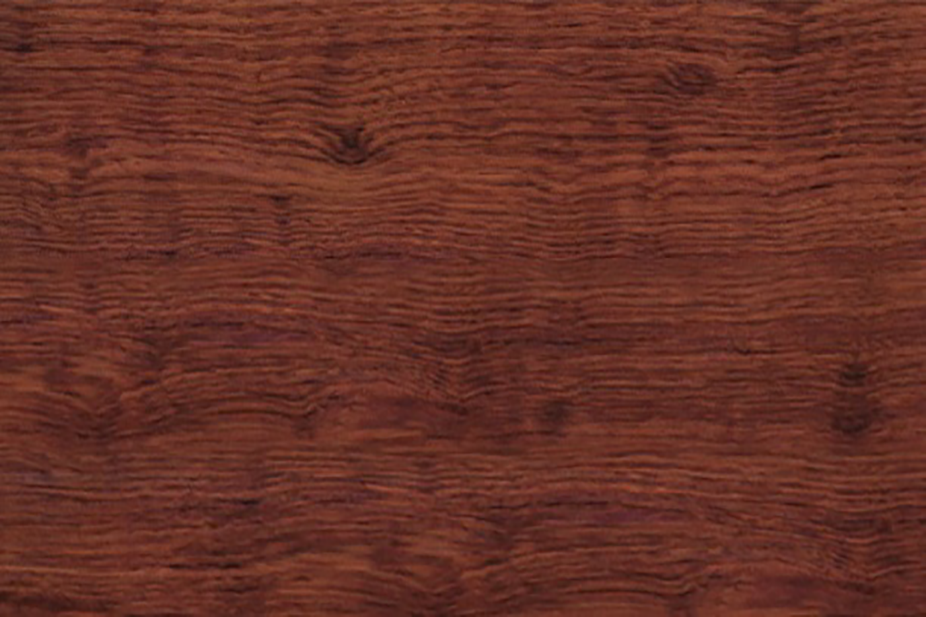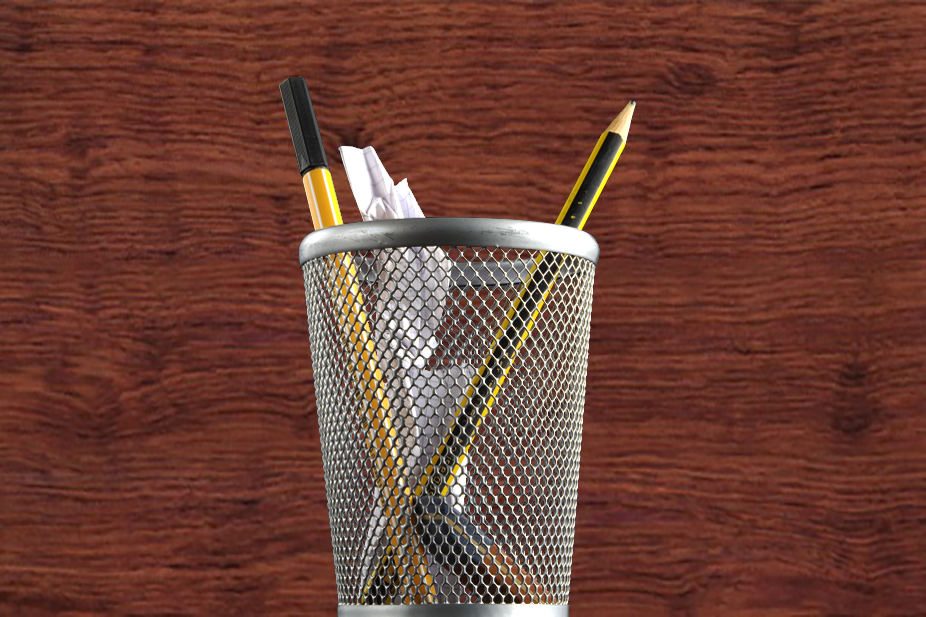img = imread('b.png');
%R通道
R = double(img(:,:,1));
%G通道
G = double(img(:,:,2));
%B通道
B = double(img(:,:,3));
%Alpha通道
subplot(1,3,1);
imshow(P),title('要剪切的图片');
subplot(1,3,2);
imshow(background),title('背景图片');
%计算参数
a = double(Alpha)/255.00;
%三通道合成
img2(:,:,1) = uint8(R .* a + (1-a) .* double(background(:,:,1)));
img2(:,:,2) = uint8(G .* a + (1-a) .* double(background(:,:,2)));
img2(:,:,3) = uint8(B .* a + (1-a) .* double(background(:,:,3)));
subplot(1,3,3);
imshow(img2),title('合成的图片');